Next: Simple Harmonic Oscillator Up: One-Dimensional Potentials Previous: Alpha Decay

# Square Potential Well

Consider a particle of massand energyinteracting with the simple square potential well(372)

where.

Now, ifthen the particle is unbounded. Thus, when the particle encounters the well it is either reflected or transmitted. As is easily demonstrated, the reflection and transmission probabilities are given by Eqs. (327) and (328), respectively, where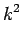(373)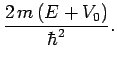(374)

Suppose, however, that. In this case, the particle is bounded (i.e.,as). Is is possible to find bounded solutions of Schrödinger's equation in the finite square potential well (372)?

Now, it is easily seen that independent solutions of Schrödinger's equation (301) in the symmetric [i.e.,] potential (372) must be either totally symmetric [i.e.,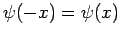], or totally anti-symmetric [i.e.,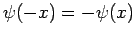]. Moreover, the solutions must satisfy the boundary condition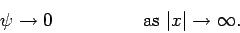(375)

Let us, first of all, search for a totally symmetric solution. In the region to the left of the well (i.e.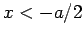), the solution of Schrödinger's equation which satisfies the boundary condition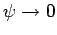and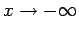is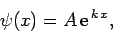(376)

where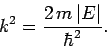(377)

By symmetry, the solution in the region to the right of the well (i.e.,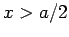) is(378)

The solution inside the well (i.e.,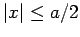) which satisfies the symmetry constraintis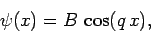(379)

where(380)

Here, we have assumed that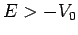. The constraint that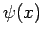and its first derivative be continuous at the edges of the well (i.e., at) yields(381)

Let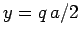. It follows that(382)

where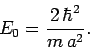(383)

Moreover, Eq. (381) becomes(384)

with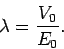(385)

Here,must lie in the range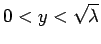: i.e.,must lie in the range.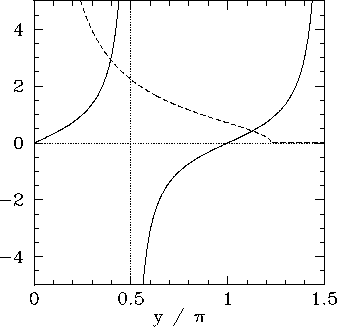Now, the solutions to Eq. (384) correspond to the intersection of the curve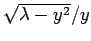with the curve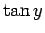. Figure 16 shows these two curves plotted for a particular value of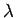. In this case, the curves intersect twice, indicating the existence of two totally symmetric bound states in the well. Moreover, it is evident, from the figure, that asincreases (i.e., as the well becomes deeper) there are more and more bound states. However, it is also evident that there is always at least one totally symmetric bound state, no matter how smallbecomes (i.e., no matter how shallow the well becomes). In the limit(i.e., the limit in which the well becomes very deep), the solutions to Eq. (384) asymptote to the roots of. This gives, whereis a positive integer, or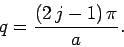(386)

These solutions are equivalent to the odd-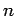infinite square well solutions specified by Eq. (307).For the case of a totally anti-symmetric bound state, similar analysis to the above yields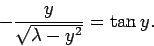(387)

The solutions of this equation correspond to the intersection of the curvewith the curve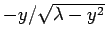. Figure 17 shows these two curves plotted for the same value ofas that used in Fig. 16. In this case, the curves intersect once, indicating the existence of a single totally anti-symmetric bound state in the well. It is, again, evident, from the figure, that asincreases (i.e., as the well becomes deeper) there are more and more bound states. However, it is also evident that whenbecomes sufficiently small [i.e.,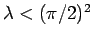] then there is no totally anti-symmetric bound state. In other words, a very shallow potential well always possesses a totally symmetric bound state, but does not generally possess a totally anti-symmetric bound state. In the limit(i.e., the limit in which the well becomes very deep), the solutions to Eq. (387) asymptote to the roots of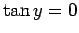. This gives, whereis a positive integer, or(388)

These solutions are equivalent to the even-infinite square well solutions specified by Eq. (307).Next: Simple Harmonic Oscillator Up: One-Dimensional Potentials Previous: Alpha Decay
Richard Fitzpatrick 2010-07-20